# Solution assignment 10 Inequalities with fractions

### Assignment 10

Solve: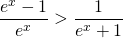### Solution

Both denominators cannot be equal to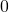for any value of. So, the inequality is valid for all.
We take the right-hand side to the left: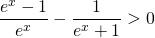We convert fractions to the same denominator and get: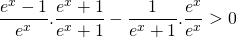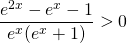The factors in the denominator are positive for all(exponential functions) and thus the inequality is valid if the numerator is greater than. Thus:The numerator in the left-hand side is a quadratic function in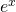and the solutions are (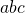-formula):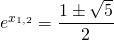The graph of the quadratic function is an 'opens up' parabola and is positive if: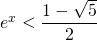or: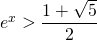The first inequality is not possible because the right-hand side is negative and the left-hand side is an exponential function which is always positive.
Thus we use the logarithm in the left- and right-hand side and get: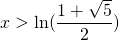0